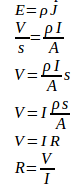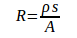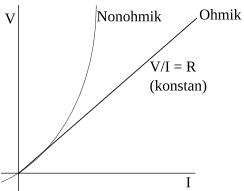# Ohm’s law

### Definition of Ohm’s law

In almost all metal conductors, the electric field is proportional to the density of the electric current, where the ratio of the electric field to the electric current density is constant. Mathematically expressed through the equation:

ρ = E / J

E = electric field, ρ = resistivity, J = current density

The constant ρ is called resistivity, whose value is constant and does not depend on the electric field that gives rise to the electric current.

If the electric field increases, the density of electric current also increases, whereas if the electric field decreases, the density of electric current is also reduced,

so that the ratio of the electric field to the density of electric current is constant. This is Ohm’s legal statement. So, the above equation explains Ohm’s law only when ρ is constant and does not depend on the electric field. Ohm’s law does not apply if the value of ρ changes when the electric field changes.

However, it is easier to measure the electric potential (V) than an electric field (E), so it is also easier to measure electric current (I) than the density of electric current (J). Therefore, in addition to being stated in the electric field, the density of electric current and resistivity (ρ), Ohm’s law is also expressed in electric potential, electric current and electrical resistance (R).

First review a wire conductor that has a length (l), cross-sectional area (A) and there is an electric potential difference (V) between the two ends of the wire. If the homogeneous electric field is all the same, then the relationship between the electric field and the electric potential difference is expressed in the equation V = E s (this equation has been discussed in electrical potential). If the density of homogeneous electric currents is the same,

then the relationship between electric current and the density of electric current is expressed in the equation I = J A (this equation has been discussed in the topic of electric current).

To obtain an equation that states the relationship between electric potential (V), electric current (I) and electrical resistance (R),

replace E and J in the equation of resistivity, with E in the equation of electric potential and J in the equation of electric current:This is an equation that explains the relationship between electric potential (V), electric current (I) and electrical resistance (R) in a conductor. Based on this equation, it can be stated that the electrical resistance in a conductor is a comparison of the electrical potential difference to the electric current in the conductor.

Ohm’s law states that the ratio between the electric potential difference to an electric current is constant. In other words, this equation explains Ohm’s law only when the electrical resistance (R) is constant. Ohm’s law does not apply if the electrical resistance is not constant.

In the above equation, the electrical resistance (R) in a conductor also has a relationship with the resistivity (ρ), conductor length (l) and cross-sectional area (A) of the conductor. Mathematically expressed in the equation:### The equation of Ohm’s law

Ohm’s law is explained by two equations, namely ρ = E/J and R = V/I. It should be remembered that these two equations are equations of Ohm’s law only when ρ is constant and R is constant. If ρ and R of a conductor changes, these two equations do not explain Ohm’s law, but ρ = E/J explains the resistance of a type of conductor and R = V/I explain the electrical resistance of a conductor.

The equation ρ = E/J can be written as E = ρ J. When ρ is constant, E is proportional to J, meaning that if E increases then J increases or if E decreases then J decreases.

Likewise, the equation R = V/I can be written as V = I R. When R is constant then V is directly proportional to I, meaning that if V increases, then I increase or if V decreases, then I decrease.

Material that obeys Ohm’s law is said to be ohmic, whereas the material that does not obey Ohm’s law is said to be nonohmic. Almost all metal conductors are ohmic, while many other objects are nonohmic.

On nonohmic objects, the electrical resistance is not constant and depends on electric current and electric potential difference. This means that if the electric current changes then the electrical resistance of the material also changes, as well as if the electrical voltage changes then the electrical resistance of the material also changes. Because of the electrical resistance changes than in the nonohmic matter, the electric voltage (V) is not directly proportional to the electric current (I).

Graph V, I and RIn ohmic matter, the ratio V to I is constant, which is represented by a straight line. An example of the ohmic material is a metal conductor. Whereas in the nonohmic matter, the ratio of V to I is not constant, which is represented by a curved line. Examples of nonohmic material are semiconductor diodes and transistors.

Ohm’s law only applies to ohmic material and does not apply to all matter, so it is inappropriate to say laws such as Newton’s law or other physical laws. The physical law actually applies to all objects, not only for certain objects. But the mention of Ohm’s law has become a habit, so it is still called Ohm’s law, provided that it is remembered that Ohm’s law only applies to ohmic objects.